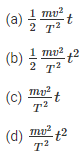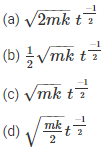Knowledge

# Work, Energy and Power MCQ for NEETWritten by

NEET Physics is the scoring paper in medical entrance examination. Here, you will get NEET Physics MCQ questions for all concepts as per the latest syllabus. Practice more on a regular basis with these NEET Physics objective questions on Air Pollution and improve your subject knowledge and problem-solving skills along with time management. NEET Physics Work, Energy and Power multiple choice questions make you feel confident in answering the question in the exam and increase your score to higher.

## MCQs on Work, Energy and Power

1. How much will the kinetic energy increase if the momentum is increased by 20%?
(A) 55%
(B) 66%
(c) 44%
(d) 77%

2. Calculate the energy loss in a perfectly inelastic collision if the mass of the object is 40 kg and velocity is 4 m/s and it collides with an object of mass 60 kg with velocity 2 m/s. Collides.
(a) 440 J
(B) 110J
(c) 392J
(d) 48 J

3. Calculate the final kinetic energy of a block of any mass if the mass of the block is 10 kg and its constant velocity is 10 m/s. A retarding force of F=0.1J/m is applied on a block of mass
(a) 275 J
(B) 250J
(c) 475 J
(d) 450 J

4. What is the average power required to lift a mass of 100 kg to a height of 50 m in 50 seconds?
(A) 980
(B) 100
(c) 50
(d) 5000

5. What is the power of the engine when the velocity of the car is v, mass m, acceleration a and external resistance R
(A) (R-MA)V
(B) (R+MA)V
(c) maw
(d) RV

6. Consider an object whose mass m is such that it is uniformly accelerated from rest and let v be the speed attained by the object in time T. Calculate the instantaneous power imparted to the body in terms of work of timeAnswer: (B) $$\frac{1}{2} \frac{mv^{2}}{T^{2} 7. A ball is thrown vertically downwards from a height of 20 m and its initial velocity is \(v_Education^{2}$$. As soon as the stone hits the ground, 50% of its energy is lost and bounces back to the same height. What is the initial velocity?
(a) 28 m/s
(b) 20 m/s
(c) 10 m/s
(d) 14 m/s

8. Consider a particle of mass m which is driven by a machine whose constant power is k watts. If the particle starts from rest then what is the force acting on the particle at time t?Answer: (d) $$\sqrt{\frac{mk}{2}} t^{\frac{-1}{2}}$$

9. When can one say that work is done on the body?
(a) When the body experiences a force
(b) When energy increases due to mechanical effect
(c) When the body moves a certain distance
(d) none of the above

Answer: (b) When energy increases due to mechanical effect

10. Select the incorrect statement for the given situation: Two bodies of mass m each.1 and m2 undergo elastic collision
(a) when m1 and m2 is at rest, the kinetic energy transferred will be maximum
(b) If M then the bodies will move in the same direction1 = m2 And when the collision is oblique
(c) when m1 >M2M2 will move four times faster than m1
(d) when M1 < m2The transfer of momentum will be maximum

Answer: (c) when M1 >M2M2 will move four times faster than m1

11. What is the ratio of velocities of a nucleus which splits into two parts with radius 1:2?
(A) 6:1
(B) 2:1
(c) 8:1
(d) 4:1

12. What is the relationship between E, P and E if E is kinetic energy, P is momentum and V is the velocity of the particle?Answer: (d) $$P=\frac{d E}{d V}$$